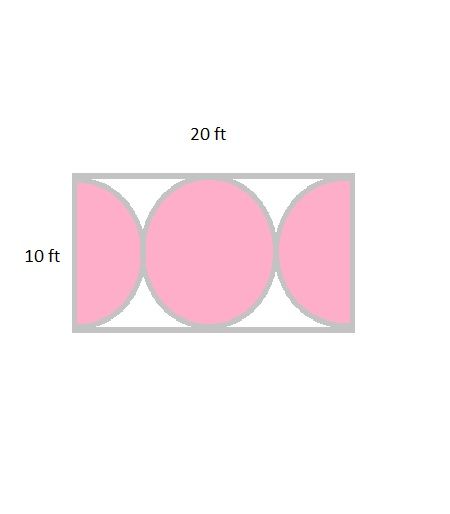# Mr. Petri has a rectangular plot of land with length = 20 feet and width = 10 feet. He wants to...

## Question:

Mr. Petri has a rectangular plot of land with length = 20 feet and width = 10 feet. He wants to design a flower garden of o circle with two semicircles at each end of the center circle. he will fill in the shaded region with wood chips. if one bag of wood chips cover 5 square feet, how many bags must he buy?

## Area of the Shaded Region:

In the rectangular plot, we can identify two circles. So, the diameter of the circle is {eq}10 {/eq} feet and the radius is {eq}5 {/eq} feet. The area of rectangle is {eq}l \times w {/eq} square units. And the area of the circle is {eq}\pi r^{2} {/eq}. The area of the shaded region is found by subtracting the area of two circles from area of rectangle.

Let us consider the rectangular plot diagram:{eq}\begin{align*} \text{Area of white shaded region} &= \text{Area of rectangle} - \text{Area of circle} \\ \\ \text{Area of rectangle} &= l \times w \\ &= 20 \times 10 \\ &=200 ft^{2} \\ \\ \text{Area of two circles} &= 2(\pi r^{2}) \\ &=2(\pi (5)^{2}) \\ &=2(25 \pi) \\ &=157.1 ft^{2} \\ \\ \text{Area of white shaded region} &= 200 - 157.1 \ ft^{2} \\ &= 42.9 \ ft^{2} \\ \\ \text{Finding number of bags}: \\ \text{One bag covers } \ & 5 \ ft^{2} \\ \text{So}, \\ &=\frac{42.9}{5} \\ &=8.58 \end{align*} {/eq}

Therefore, Mr. Petri needs to buy totally {eq}9 {/eq} bags of wood chips to fill the shaded region.